# Year 4 Maths Task- Monday 23rd March

Tenths and Hundreths

You might need to work out on paper and then write it in your reply. If you have a printer, you could print it out and write your answers on there first.

This activity should take around an hour.

Click on the blue link to see the work more clearly.

maths lesson 1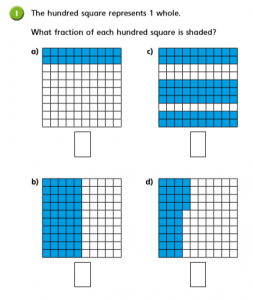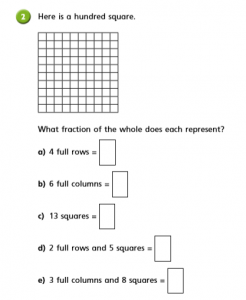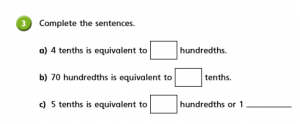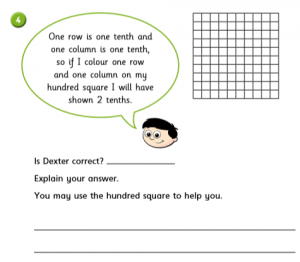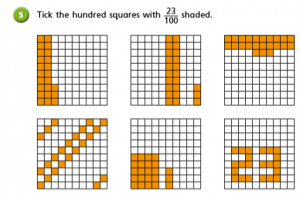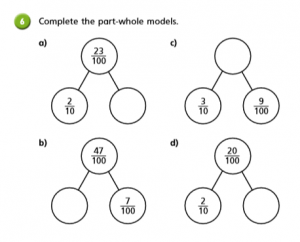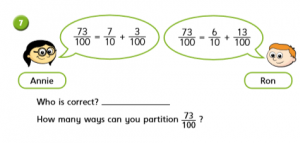### 18 thoughts on “Year 4 Maths Task- Monday 23rd March”

•23rd March 2020 at 11:43 am

1. A. 20/100=5/25
b. ½
c. 70/100=7/10
d. 34/100

2.
a. 4 full rows = 40/100=4/10
b. 6 full rows = 60/100=6/10
c. 13 squares= 13/100
d. 2 full rows and 5 squares =25/100
e. 3 full colomns and 8 squares = 38/100

3. A. 4 tenths is equivalent to 40 hunderdths
b. 70 hundredths is equivalent to 7 tenths.
c. 5 tenths is equivalent t0 50 hunderedths and half

4. This is incorrect because he is used the first square to make the next column.
5. A,B,C,D and F.
6. A. 3/100
b. 4/10
c. 39/100
d. 0/100
7. Both are correct because they have partitioned in different ways.

•23rd March 2020 at 12:07 pm

Fantastic understanding of tenths and hundredths Brooke. well done

•23rd March 2020 at 11:51 am

1. A. 2/10
b. ½
c.7/10
d.34/100

2.a. 40/100=4/10
b. 60/100=6/10
c 13/100
d 25/100
e 38

3.
a. 4 tenths is equivalent to 
b 70 hundeths is a equivalent to  tens
c. 5 tenth is equivalent to  hunderths or 1 half

4. He is incorrect because he has used the first square

5. a b c d e f g

6. 23/100 2/10 3/100
39/100 3/10 9/100
47/100 4/10 7/100
20/100 2/10 0/100

•23rd March 2020 at 12:10 pm

You have a great knowledge of tenths Samuel, well done

•23rd March 2020 at 11:56 am

1. A. 20/100 =2/10
b.50/100=5/10
c.70/100=7/10
d. 34/100
2. A. 4 full rows=40/100=4/10
b. 6 full rows=60/100=6/10
c. 13 squares =13/100
d. 2 full rows and 5 squares=25/100
e. 3 full columns and 8 squares=38/100
3. A. 4 tenths is equivalent to 40
b. 70 hundredths is equivalent to 7 tenths.
c. 5 tenths is equivalent to 50 hundreds or 1 half.
4. Is Dexter correct ? No he is not correct because he uses one of the squares in the column. #
5. a,b,c,e and f
6. a. 3/100
b. 4/10
c. 39/100
d. 0/100
7. Annie because they are partitioning 7 tenths and 3 hundedths.

•23rd March 2020 at 12:09 pm

Great understanding of hundredths and tenths Bella

•23rd March 2020 at 12:05 pm

1.a. 2o/100=2/10
b. ½
c. 70/100
d.34/100
2.a 4 full rows =40
b. 6 full colloms =60
c. 13 squares =13
d. 2 full rows and 5 squares =25
e. 3 full colloms and 8 squares =38
3. a 4 tenths is equivalent to 50 hundredths
b. 70 hundredths is equivalent to 7 tenths

•23rd March 2020 at 12:09 pm

Great job Maisie!

•23rd March 2020 at 2:00 pm

1: a = 1/5, b = 1/2, c= 7/10 d= 17/50

2: a= 4/10, b= 6/10, c= 13/100, d= 1/4, e = 38/100

3: a: 40/100, b= 7/10, c= 50/100 or 1/2

4: Dexter is not correct. When he coloured in his column he had already coloured in one square for a row. Correct answer was 19/100

5: 1 to 5 have 23 squares, 6 doesn’t

6: a= 3/100, b= 4/10, c= 39/100, d= 0/100

7: Both are correct

5/10 + 23/100
4/10 + 33/100
3/10 + 43/100
2/10 + 53/100
1/10 + 63/100

•23rd March 2020 at 2:53 pm

Fantastic job on your maths Owen, working out equivalent fractions as well which is great.

•24th March 2020 at 3:08 pm

1
a)20/100
b)50/100
c)70/100
d)34/100

2.
a)4 full rows=40/100
b)6 full rows=60/100
c)13 squares=13/100
d)2 full rows and 5 squares=70/100
e)3 full columns and 8 squares=38/100

3.
a)4 tenths is equivalent to= 4 hundredths
b)70 hundredths is equivalent to=7 tenths
c)5 tenths is equivalent to=5 hundredths or 1 hudredths

4.
yes/ he’s correct because 2times10=20 so 2 tenths

5.
4 correct=23/100

6.
a)23/100-2/10+21/100
b)47/100-40/10+7/100
c)39/100-3/10+9/100
d)20/100-2/10+18/100

7
annie

73/=7/10+3/100
73/100=3/100+7/10=commutative

•25th March 2020 at 12:10 pm

Fantastic Kristers. It’s great to see all your hard work on the blog!

•26th March 2020 at 4:23 pm

1a) 20/100= 2/10
b) 50/100
c) 70/100= 7/10
d) 34/100
2a) 40/100= 2/5
b) 60/100= 3/5
c) 13/100
d) 25/100= 1/4
e) 38/100
3 a) 40 hundredths
b) 7 tenths
c) 50 hundredths
4) He is incorrect. A row and a column will always cover one square together.
5) All except last one (number 6).
6a) 3/100
b) 39/100
c) 4/10
d) 0/100
7) Annie

•28th March 2020 at 6:35 pm

1.
a. 20/100=2/10=1/5
b. 50/100=1/2
c. 70/100=7/10
d. 34/100

2.
a. 4 full rows = 40/100=4/10
b. 6 full rows = 60/100=6/10
c. 13 squares= 13/100
d. 2 full rows and 5 squares =25/100=1/4
e. 3 full columns and 8 squares = 38/100

3.
A. 4 tenths is equivalent to 40 hundredths
b. 70 hundredths is equivalent to 7 tenths.
c. 5 tenths is equivalent to 50 hundredths and 1half

4.
This is incorrect. 1 column and 1 row will make 19 squares, not 20.
5.
A,B,C,D and E.
6.
a. 3/100
b. 40/100
c. 39/100
d. 0/100
7.
Both are right.
73/100=50/100+20/100+3/100=1/2+2/10+3/100
73/100=4/10+33/100

•29th March 2020 at 3:08 pm

Well done, Alex! I can see you’ve read the questions carefully.

•1st April 2020 at 2:07 pm

1.
a)2/10
b)5/10
c)7/10
d)34/100

2.
a) 4/10
b) 6/10
c) 13/10
d) 25/100
e) 38/100

3.
a) 4
b) 7
c) 50 or half

4. No
Dexter is wrong because there are 10/10 and 9/100

5) all but last

6)
a) 3/100
b) 4/10
c)39/100
d)0/100

7) Both are correct
7 ways

•1st April 2020 at 2:45 pm
•